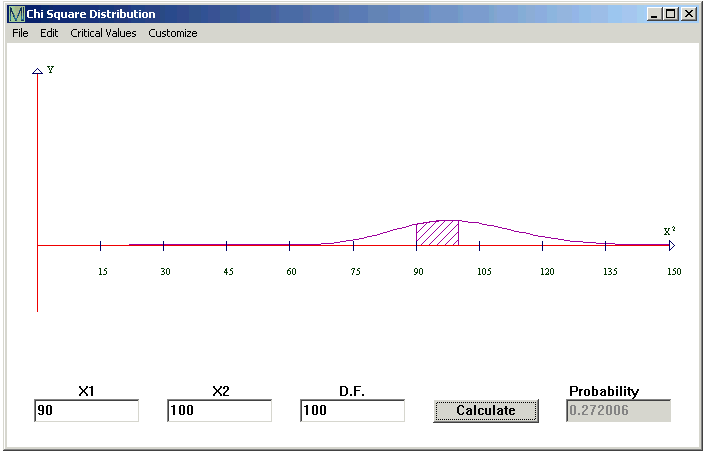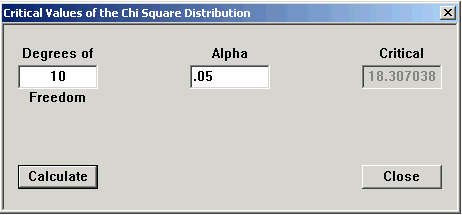# Chi Square Distribution

The Chi Square Distribution is commonly used for data that is squared, to test Standard Deviation or Goodness of Fit. Because it is not negative, the graph is a distorted bell curve. The middle of the curve is not at zero.

CalGraph will calculate the chance under the chi square curve, that is, the probability that the variable lies between two values. This variable is usually written c2

Click on Statistics at the top of the window and then on Chi Square Distribution. This window opens.Type the lower number in the first box, the higher number in the second box and the Degrees of Freedom in the D.F. box. Then click Calculate.

Here CalGraph shows that the chance the Chi Square variable with 100 Degrees of Freedom is between 90 and 100 is 0.272006. The graph shows the area under the curve shaded.

You may want the area below some value, say  below 60. Because Chi Square is always positive, this means from 0 to 60. Put 0 in the X1 box and 60 in the X2. Hit Calculate.

Again, you might want the area above some value, maybe above 80.   This means chi square runs from 80 to infinity. Put 1000 (or any number above 1000) in the second box. Don't forget to type in the Degrees of Freedom. I know, 1000 is not infinity, but to 6 decimal places, there is no difference in the result.

You can print the graph and your result by clicking File and then Print. Or you can copy it to another document, using Edit > Copy Graph to Clipboard and then Paste in that document.

CalGraph will also calculate the other way,

Given an area, CalGraph will find the Critical Value which cuts off that area. At the top of the Chi Square Distribution window, click on Critical Values and then Alpha.Type in the Degrees of Freedom. Type the area you know in the alpha box and click Calculate.

Here using 10 Degrees of Freedom, 0.05 is the area of the region under the curve between 18.307038 and infinity.

CalGraph will calculate the p-value for a hypothesis test.

CalGraph will not calculate Chi Square if the Degrees of Freedom is more than 280.